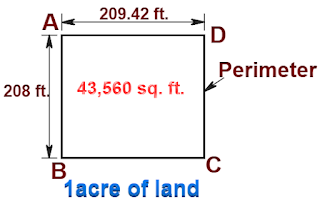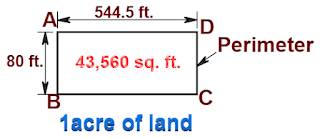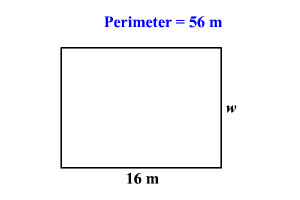# What will be the Cost of Boundary Fencing Per Acre | Metal Fence Estimate:

In this Article today we will talk about the What Will be the Cost of Boundary Fencing | Fence Cost Estimator | Fence Cost Calculator | Fencing Cost Per Acre | How to Calculate Cost of Fencing | Metal Fence Estimate

## What is Fence?

fence is a structure that encloses an area, typically outdoors, and is usually constructed from posts that are connected by boards, wire, rails or netting. A fence differs from a wall in not having a solid foundation along its whole length. Fence Cost Estimator

## How Much Does It Cost to Fence One Acre of Land?

Now, let us find out the answer for one of the FAQs “What will be the cost of boundary fencing per acre?

My response is, This is a non-answerable wrong question.

The reason is, the area of any land does not define its perimeter. The cost differs from one land to another having the same measured area.

I will illustrate this with the following 2 examples. Fence Cost Estimator

### 1. Land no 1:#### Given data:

Length of  side AB = CD = 208 ft.

Length of side AD = BC = 209.42 ft.

Area of rectangular land = [length × breadth]

= [209.42 ft. × 208 ft.]

= 43,560 sq ft. = 1 acre

[ As 1acre = 43,560 sq ft.] Fence Cost Calculator

#### Perimeter of land no 1.

= [side AB + side BC + side CD + side DA]

= [208 ft. + 209.42 ft. + 208 ft. + 209.42 ft.]

834.84 ft. How to Calculate Cost of Fencing

### 2. Land no 2:#### Given data:

Length of  side AB = CD = 80 ft.

Length of side AD = BC = 544.5 ft.

Area of rectangular land = [length × breadth]

= [544.5 ft. × 80 ft.]

= 43,560 sq ft. = 1 acre

[ As 1acre = 43,560 sq ft.]

#### The perimeter of land no 2.

= [side AB + side BC + side CD + side DA]

= [80 ft. + 544.5 ft. + 80 ft. + 544.5 ft.]

1249 ft.

Now, let us consider installing a barbed-wire fence of the same height for both types of land.

Before proceeding further, go through the article👇 Fence Cost Calculator

## Calculating the Cost of Barbed Wire Fencing for the Land:

Let us find out the material & labor cost for barbed wire fencing for the land.

### Material cost:

Sl.No.       Description        Quantity      Rate      Unit      Amount

1.   RCC fencing poles            40.0           270         no.     10,800.00

2.   Boulder for packing        0.42417       1400       cum        593.83

3.   Cement bags for

concreting                             6.837         400        bag      2734.80

4.    Sand for concreting        0.3563       2200       cum.     783.86

5.    Aggregate for

concreting                           0.4751         1850       cum.     878.93

6.   Barbed wire for

fencing                              37.88             150           kg.     5682.00

Total material cost for fencing =  INR/Rs  21,473.42

### Labor cost:

Sl.No.   Description        Quantity      Rate      Unit      Amount

1.   Earthwork excavation

for the fencing pole           1.27251          800    cum         1017.60

2.    Installation and

concreting the pole               40              120      no.          4800.00

3.  Tying fencing wire            840              3      meter         2520.00

Total labor cost for fencing = INR/Rs  8337.00

The total cost of fencing the land = [total material cost + labor cost]

= [ 21473.42 + 8337]

INR/Rs.  29810.42

Cost per running ft.

= [total cost ÷ perimeter of the land in ft.]

= [29810.42 ÷ 260 ft.]

=  114.65/R.FT.

= 376.64 / running meter. How to Calculate Cost of Fencing

### 1. Cost of fencing for land 1:

= [ perimeter  × fence cost/ r ft.]

= [ 834.84 ft.  × 114.65/-]

INR 95,714.4/-

### 2. Cost of fencing for land 2:

= [ perimeter  × fence cost/ r ft.]

= [ 1249 ft.  × 114.65/-]

= INR 1,43,197.85/-

Note: The fencing cost / r ft. is taken from the above-linked article.

#### Difference in Cost:

The difference in the fencing cost of land 1 & land 2

= [1,43,197.85 – 95, 714.4]

47,483.45/-

i.e. whopping 49.6% difference in the fencing cost of the 1-acre of land having different dimensions. I hope you understood why we cannot answer this question

## FAQs:

### Q. How Do You Calculate the Area of a Fence?

Example:

The perimeter of a rectangular pool is 5656 meters. If the length of the pool is 1616 meters, then find its width.

Here the perimeter and the length of the rectangular pool are given. We have to find the width of the pool.The perimeter PP of a rectangle is given by the formula, P=2l+2wP=2l+2w , where ll is the length and ww is the width of the rectangle.

Given that, the perimeter is 5656 meters and the length is 1616 meters. So, substitute these values into the formula.

56=2(16)+2w56=2(16)+2w

Simplify.

56=32+2w56=32+2w

Subtract 3232 from both sides.

24=2w24=2w

Divide each side by 22 .

12=w12=w

Therefore, the width of the rectangular pool is 1212 meters.

### Q. What is the Cost of Fencing Per Meter?

Fencing is typically charged on a per metre basis and could range from $60 to$1,200 per meter. This figure will typically include labour for installation and the cost of materials. Ideally, a contractor will visit your property to provide an accurate quote.

### Q. What will be the cost of fencing rectangular?

Rate of the wire is Rs20 per meter. Hence, the total cost of fencing the rectangle compound is Rs 536. So, the correct answer is “536 Rs”.

### Q. What is the distance between fence posts?

between 8-12 feet

How far apart should fence posts be? In general, T-posts are spaced between 8-12 feet apart, depending on what you’re trying to contain or keep out. Closer spacing is suggested for large livestock, like cattle, while wider spacing is fine for fending off deer and other large pests

### Q. How do you calculate how many fence posts do I need?

Number of Posts: As we said earlier, posts should be no longer than 8 feet apart from each other. Some homeowners go with 16 feet, but if you do, your fence will not be as sturdy. If you go with 8 feet, divide your fence length by 8 to get the total number of posts. Therefore, a 100-foot fence would require 12 posts.

## Conclusion:

Full article on What Will be the Cost of Boundary Fencing | Fence Cost Estimator | Fence Cost Calculator | Fencing Cost Per Acre | How to Calculate Cost of Fencing | Metal Fence Estimate. Thank you for the full reading of this article in “The Civil Engineering” platform in English. If you find this post helpful, then help others by sharing it on social media. If any formula of BBS is missing from this article please tell me in comments.

1.Khursheed

Great

• Thanks for the comments please visit more articles by clicking on “All Posts” tab of the Menu Bar

2.engineering geeks

awesome

• Thanks for the comments please visit more articles by clicking on “All Posts” tab of the Menu Bar

3.Engineering Geeks

A fence is necessary to keep the privacy of any land. It keeps out the intruders who may otherwise encroach upon the land. It is a good thing even for neighboring houses. The Neighbours might be friendly towards each other.